## //----------------------------// ArtMatic 4 in 1 out components //----------------------------//

Introduction
Ax+By+Cz+Dw
Multiply
Determinant
Dot product (U,V)
Pack
Min(x,y,z,w)
Max(x,y,z,w)
Distance(U,V)
DF Curve
DF 'S' Curve
Bezier Curve
Random Mix
4D Perlin noise
3D w Fractal noises #
DEM Mandelbrot(U*U+V)
2D Motion Path render
3D Motion Path render
41 Compiled tree

## Introduction

41 Components have three main uses: to mix four independent outputs, to create a texture from two space transforms, and to pack the outputs of RGB+Alpha components into a stream to be fed to a packed mixer. They also can be a 4D scalar field generator.

An essential in-depth discussion of ArtMatic structures Trees and components is found in ArtMatic Designer References and in Building trees.

##41 Ax+By+Cz+Dw

parameters :
A : Scale X (-8. : 8.)
B : Scale Y (-8. : 8.)
C : Scale Z (-8. : 8.)
D : Scale W (-8. : 8.)

discussion :
This component is used to sum (mix) 4 input values. Parameters A, B and C and D are applied as scaling factors to the (x,y,z,w) inputs prior to summing the values. It is basically a 4D plane equation where (A,B,C,D) is the plane normal vector.

##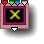41 Multiply

parameters :
A : Amplitude (0. : 2.)
B : Offset (-4. : 4.)

discussion :
Multiply the input values together. Amplitude (parameter A) scales the output, and Offset (parameter B) is added to the result.

##41 Determinant

parameters :
A : Amplitude (0. : 2.)
B : Offset (-4. : 4.)

discussion :
Mathematical primitive that returns the determinant of the 2D matrix ((x,y),(z,w)) that is x*y-z*w. Result is scaled by 'Amplitude' and the Offset B is added.

##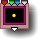41 Dot product (U,V)

parameters :
A : Amplitude (-8. : 8.)
B : Offset (-8. : 8.)

discussion :
Mathematical primitive that computes the
dot product of vectors U (xy) and V (zw) i.e. x*z + y*w. This can be used to find the distance to a line. If the vectors are normalized, the dot product is the cosine of the angle between U and V. To explicitly find that angle you may use the acos function available in 11 Math tools #

algorithms :
• U.V :
• Normalised(U.V) :

##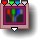41 Pack

parameters :
No parameters.

discussion :
Pack the four inputs into a single stream. The output should be fed to a component that expects packed input (such as the packed mixers). The use of the pack component allows to send several streams of RGBA datas to various packed mixer components.
You can also pack 3 values with
Pack(x,y,z) .
Note that in ArtMatic Engine 8.06 tiles that don't take packed will now implicitely use the 4th (alpha or DF) value instead of red when connected to Packed RGBA outputs. This applies also for inputs connected to CT that outputs packed RGBA.

##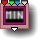41 Min(x,y,z,w)

parameters :
A : Amplitude (0.50 : 1.50)

discussion :
Compare the four inputs (x, y, z and w) and pass out the minimum value multiplied by Amplitude (parameter A).

##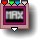41 Max(x,y,z,w)

parameters :
A : Amplitude (0.50 : 1.50)

discussion :
Compare the four inputs (x, y, z and w) and pass out the maximum value multiplied by Amplitude (parameter A).

##41 Distance(U,V)

parameters :
A : Amplitude (-8. : 8.)
B : Offset (-8. : 8.)

discussion :
Mathematical primitive that computes the 2D distance from vector U(xy) to V(zw) or Length(U-V). The result can be further scaled by 'Amplitude' and translated by 'Offset'. Use 1 & 0 for the straight euclidian distance between U & V.

##41 DF Curve

parameters :
A : Angle A (-45. : 45.)
B : Angle B (-90. : 90.)
C : Curvature % (0. : 1.)

discussion :
Uses various smooth logic operations to generate a DF curve between two slopes. Angle A & B defines the directions while the "Curvature" sets the smoothness and extent of the curve.
Input 3 & 4 (z,w) should be connected to a 12 constant tile while x and y input are typically connected to a 2D space.
Parameters on input 3 and 4 (z,w) are used to translate the 2 lines defining the curve. They should be constants otherwise result won't be DF compliant.

41 DF Curve has 3 algo : Concave, Convex and Concave + Y clipped.

DF Curve provides lots of application for graphic design, decorative art and 3D modeling. Since in 3D it will create an infinite curved plane, the functions needs to be mixed with other 3D primitives to create a bounded object.##41 DF 'S' Curve

parameters :
A : Angle A (0. : 45.)
B : Angle B (-90. : 0.)
C : Curvature % (0. : 1.)

discussion :
Uses various smooth logic operations to generate an S curve between two slopes. Angle A & B defines the directions while the "Curvature" sets the smoothness and extent of the curve.

The offset of each lines can be passed as parameters on input 3 and 4 (z,w), they should be constant typically otherwise result won't be DF compliant.

DF 'S' Curve provides lots of application for graphic design, decorative art and 3D modeling.

Since in 3D DF 'S' Curve will create an infinite curved plane, the functions needs to be mixed with other 3D primitives to create a bounded object.##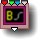41 Bezier Curve

parameters :
A : Frequency (0. : 2.)
B : Start value (-2 : 2)
C : End value (-2 : 2)

discussion :
Reimplemented in ArtMatic Engine 8.03, Bezier Curve is now DF compliant and works in a similar way as DF curves. Parameter B & C sets the height of the bezier curve end points with x coordinate being set at -2 & 2. Before and after the end point straight lines will continue the curve. Input 3 & 4 (z,w) should be connected to a 12 constant tile while x and y input are typically connected to a 2D space. z & w provided by the 2D vector constant will define the slope at start and end points given by parameters B & C. At (z,w) =(0,0) the slope is flat. When z and w are of the same sign the curve is usually convex. The curve is bounded by a large disc but is generally combined with other primitives using logic operators. if (z,w) are not connected to a constant value the input is ignored and (0,0) is used. It is often useful to rotate it by connecting the x & y to a rotation tile

##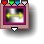41 Random Mix

parameters :
A : Amount (0. : 2.)
B : Phase (-32. : 32.)
C : Frequency (0. : 16.)

discussion :
Random crossfading of the four input values interpreted as 4 terrains elevations or surfaces. Internally Random Mix uses 2 low frequency Perlin noises to blend the 4 surfaces. See
21 Perlin Noise function.

This component is especially useful for creating terrains for ArtMatic Voyager or other complex textures.

##41 4D Perlin noise

parameters :
A : Amplitude (-4. : 4.)
B : Phase (-32. : 32.)
C : Frequency (0. : 8.)

discussion :
A 4D implementation of the Perlin Noise. Connect the fourth input to Global Input time to animate the texture in 3D automatically over time. The 4D version can be seen as a 3D noise like
3D Perlin Noise that can be animated over time as the 3D version can also be used as a 2D noise animated over time.

Interesting textures can be created by feeding this component the output of two different space transforms. You can also feed the zw input a 12 Circular motion to create a seamless looped animation of a 2D Perlin noise.

##41 3D w Fractal noises #

parameters :
A : Amplitude (0. : 8.)
B : Phase (-32. : 32.)
C : Frequency (0. : 32.)

algorithms :

##41 DEM Mandelbrot

parameters :
A : Amplitude (1. : 16.)
B : Slope (1. : 16.)
C : Iterations (4 : 2048)

discussion :
This component implements the
Distance Estimation method of calculating Mandelbrot and Julia sets. The distance estimation method provides improved continuity and accuracy compared to the standard method of calculating them (level sets).
Mandelbrot Equation is (U*U+V) where U & V are complex numbers. Typically, one pair of inputs is fed by a 12 Set Constant component.
For Mandelbrot sets feed the first input pair, vector U (x+iy) and set V (z+iw) with (0,0).
For Julia sets, use constants vector V and set U to (0,0) By animating the set of constants you can create a continuous blend from Mandelbrot sets to Julia sets

##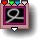41 2D Motion Path render

parameters :
A : Iterations (2 : 500)
B : step divide (1. : 256.)
C : level/width (0. : 8.)

discussion :
This component will efficiently render a large number of 2D points provided by a 12 motion function connected to input 3 & 4. The motion defining function can be a 12 tile like
Circular motion or a 12 Compiled Tree holding a time dependent function. The huge gain of speed is mostly due to the fact that the entire path is computed only once per frame instead as for every pixels using traditional ArtMatic iterative technics. To help understand and design a parametric equation you might use wolfram alpha parametric plot tool.
Input 1 and 2 (x,y) takes the plane coordinates and defines the rendering space.
When using a Compiled Tree, the CT that computes the points positions shall return the coordinates as a function of time only in a form of a parametric curve (X=F(t),Y=G(t)). The number of 'Iterations' will decide how many points are generated. The 'step divide' controls the time resolution, hence the amount of time between two consecutive points. The lower the more points will be spaced. 'level/Width' parameter sets the size of the rendered discs or line.

The output is DF compatible when the input space (x,y) is unscaled and an immense variety of modeling possibilities for 3D rendering in Voyager opens with this new component. It can be further manipulated to assign colors or to be part of a 3D model structure.

The tile has several algorithms offering various rendering options :

algorithms :
• Render as dots:
Each point is rendered as a DF sphere or a DF disc when in 2D.
• Render as DF lines:
The list of point is rendered as DF lines.
The list of point is rendered as DF lines going toward origin : (the line start at P(n)*0.5 an goes to P(n+1))
The list of point is rendered as DF lines starting at origin (0,0)
• as Morphed spheres:
The list of point is rendered as DF spheres accumulated using exponentials with the Morph algorithm explained in detail in the 11 Memory Maths "Morph" section. This mode will blend each sphere naturally and is an ideal choice for modeling clouds, smoke or fluid motions. .

Note: No other input than time shall be used within the CT as the points are computed just once before each frame and globals inputs x, y, z don't exist yet.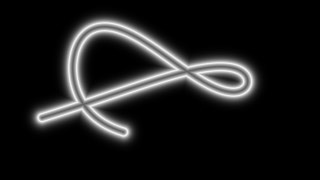A simple 2D motion path DF line 'neon'-rendered

Examples : Libraries/Maths/Parametrics & Motion/Motion 2D path neon

##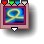41 3D Motion Path render

parameters :
A : Iterations (2 : 500)
B : step divide (1. : 256.)
C : level/width (0. : 8.)

discussion :
Similar to 2D Motion path render, 3D Motion Path render will efficiently render a large number of 3D points provided by a 11 Compiled Tree connected to input 4. It takes a 3D space in inputs (x,y,z) and the list of 3D points defining the path as a 3D or 4D packed vector in input 4 (The positions of 3D point has to be packed so they can be fed to the single input 4.)
The CT that computes the points positions shall return the coordinates as a function of time only in the form of a parametric curve (x=F(t),y=G(t),z=H(t)). When the 4th input is a 4D vector the additional information can modulate the radius of each point.
The output of the tile is a scalar DF field that can be further manipulated to assign colors or to be part of a 3D model structure.
Another version outputs local coordinates and can be seen at
3D Motion Path render
parameters:
The number of iteration will decide how many points are generated. When the CT is connected to time, points will move automatically. No global x or y inputs shall be used within the CT as the points are computed just once before each frame and x, y doesn't exist yet. The 'level/width' sets the radius of the line. The 'step divide' parameter controls the time resolution, hence the amount of time between two consecutive points which is equivalent to their spacing. Use 'Frequency' at 1 unless you want to enlarge or reduce the whole rendered shape. The output is DF compatible when the input space (x,y) is unscaled and an immense variety of modeling possibilities for 3D rendering in Voyager opens with this new component.

The tile has several rendering algorithms that offers the similar rendering options as the 2D version above.
algorithms :

• Render as dots:
Each point is rendered as a DF sphere or a DF disc when in 2D.
• Render as DF lines:
The list of point is rendered as DF lines.3D motion path rendered with 500 iterations in DF lines mode and a 'level/width' at 0.6'

The list of point is rendered as DF lines going toward origin : (the line start at P(n)*0.5 an goes to P(n+1)).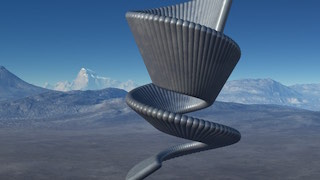3D helix motion path rendered in 'Radial half' mode.

• Normal DF lines:
The list of point is rendered as DF lines normal (orthogonal) to the path tangent and passing trough each point.3D helix motion path rendered in 'Normal' mode.

• as morphed spheres:
The list of point is rendered as DF spheres accumulated using exponentials with the Morph algorithm explained in detail in the 11 Memory Maths "Morph" section. This mode will blend each sphere naturally and is an ideal choice for modeling clouds, smoke or fluid motions. It is significantly slower than the first mode and shall be used when the motion function setting is done.3D motion path rendered in 'morphed' mode as ArtMatic volumetric unbounded clouds.
Voyager Examples/Components/DF Motion path/MetaBalls upflow s-cloud.vy

3D Examples : Voyager Examples/VY5 Animations/MetaBalls.vy, Voyager Examples/Components/DF Motion path/

## 41 Compiled Tree :

parameters :
A : Scale 0:1
B : Iterations

discussion :
Compiled trees are groups of tiles that can be used in place of single tiles as a kind of macro or subroutine.
41 compiled tree can hold any 4 inputs / 1 outputs tree structures and can be seen as a scalar 4D function unless the output is a packed vector. It can also be used for Operations on two 2D vectors that returns a single value like the dot product.